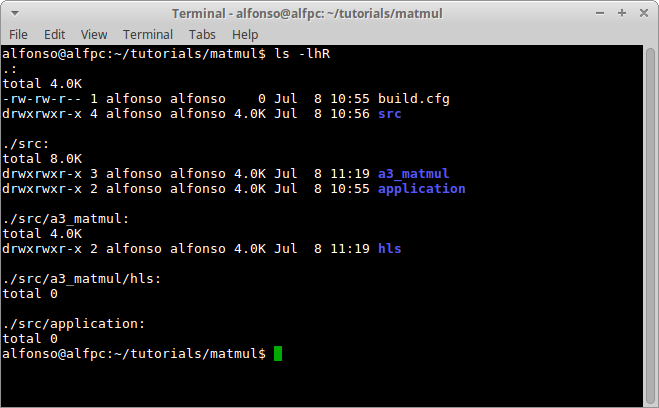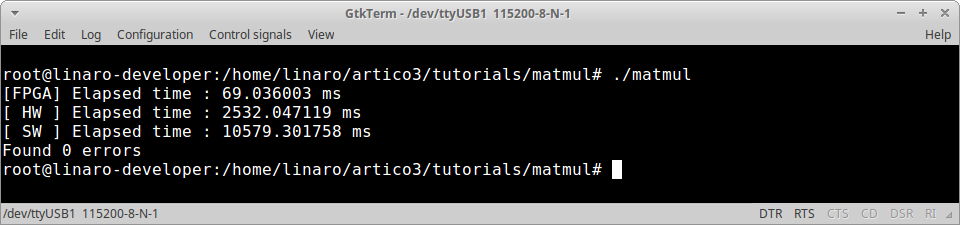# ARTICo³ – Hello, World!

Tutorial created on July 8, 2019 by A. Rodríguez

In this tutorial, you will use a block-based algorithm to multiply 512x512 matrices (32-bit unsigned integer) using accelerators capable of multiplying 64x64 matrices (32-bit unsigned integer).This tutorial covers the following topics:

• Creating and configuring an ARTICo³ project
• Describing an ARTICo³ accelerator in HLS-oriented C code
• Using the ARTICo³ runtime API

## Basic Implementation

### Set Up Workspace

The skeleton contains an empty configuration file (`build.cfg`) and the folder structure to host both application (`src/application`) and accelerator code (`src/a3_matmul/hls`). Note that, for this tutorial, you will be building a hardware accelerator using High-Level Synthesis (HLS) from C code.### Accelerator Code

Create an empty file in `src/a3_matmul/hls` called `matmul.cpp`.IMPORTANT: Although the ARTICo³ toolchain supports both C and C++ syntax, it requires file extension to be `.cpp`.

Open the file you just created and write the naive matrix multiplication algorithm for 64x64 unsigned integer matrices:

``````#include "artico3.h"
#define SIZE (64)
A3_KERNEL(a3in_t a, a3in_t b, a3out_t c) {
uint8_t i, j, k;
for (i = 0; i < SIZE; i++) {
for (j = 0; j < SIZE; j++) {
c[i*SIZE + j] = 0;
for (k = 0; k < SIZE; k++) {
c[i*SIZE + j] += a[i*SIZE + k] * b[k*SIZE + j];
}
}
}
}
``````

This code defines an ARTICo³ kernel using the `A3_KERNEL` macro. This kernel has two input ports (`a` and `b`) and one output port (`c`). In HLS-based ARTICo³ kernels, input ports are defined using the `a3in_t` label, whereas output ports are defined using the `a3out_t` label.

For more info on how to describe ARTICo³ kernels, check the documentation.

### Application Code

Create an empty file in `src/application` called `main.c`.

Write the following code skeleton:

``````#include <stdio.h>
#include <stdlib.h>
#include <sys/time.h>
#include <time.h>
#include "artico3.h"
void matmul_sw(int size, a3data_t a[size], a3data_t b[size], a3data_t c[size]) {
unsigned int i, j, k;
for (i = 0; i < size; i++) {
for (j = 0; j < size; j++) {
c[i*size + j] = 0;
for (k = 0; k < size; k++) {
c[i*size + j] += a[i*size + k] * b[k*size + j];
}
}
}
}
int main(int argc, char* argv[]) {

return 0;
}
``````

This code includes a software-based alternative to the ARTICo³-accelerated version, and will be used as a golden copy and for performance comparison.

The first thing you need to do on your application is to initialize the ARTICo³ runtime and the FPGA (i.e., load the static bitstream file). To do so, call the `artico3_init` function in `main`:

``````artico3_init();
``````

Then, you need to register a kernel in the ARTICo³ runtime by calling the `artico3_kernel_create` function in `main`:

``````artico3_kernel_create("matmul", 49152, 3, 0);
``````

This function registers a kernel called `matmul` with a local memory of 49152 Bytes symmetrically split in 3 banks, and with 0 configuration registers.

Now, hardware accelerators can be loaded in the reconfigurable slots by calling the `artico3_load` function in `main`:

``````for (i = 0; i < 4; i++) {
}
``````

This function loads the `matmul` kernel in the reconfigurable slot `i`, setting its TMR and DMR group tags to 0 (i.e., no modular redundancy enabled), and forcing the FPGA reconfiguration process (last parameter, set to 1) to remove the previous content of the slot.

Once the accelerators are available in the FPGA, shared memory buffers between application and accelerators need to be allocated. To do so, call the `artico3_alloc` function in `main`:

``````a3data_t *a = artico3_alloc(512 * 64 * sizeof *a, "matmul", "a", A3_P_I);
a3data_t *b = artico3_alloc(512 * 64 * sizeof *b, "matmul", "b", A3_P_I);
a3data_t *c = artico3_alloc(512 * 64 * sizeof *c, "matmul", "c", A3_P_O);
``````

In this function, the first argument is the size (in bytes) to be allocated for the buffer, the second is the name of the kernel to which the buffer belongs, the third is the name of the port, and the fourth is the data direction, being `A3_P_I` for inputs and `A3_P_O` for outputs. Please check the documentation for more info on port specification.

Load the input memories with data. In this tutorial, you will use randomly generated numbers:

``````// Allocate memory
a3data_t *A = malloc(512 * 512 * sizeof *A);
a3data_t *B = malloc(512 * 512 * sizeof *B);
a3data_t *C_sw = malloc(512 * 512 * sizeof *C_sw);
a3data_t *C_hw = malloc(512 * 512 * sizeof *C_hw);
// Initialize inputs
srand(time(NULL));
for (i = 0; i < (512 * 512); i++) {
A[i] = rand();
B[i] = rand();
}
``````

Implement the block-based matrix multiplication:

``````for (i = 0; i < 512; i += 64) {
for (j = 0; j < 512; j += 64) {
// Initialize accumulator
for (i2 = 0; i2 < 64; i2++) {
for (j2 = 0; j2 < 64; j2++) {
C_hw[((i + i2) * 512) + (j + j2)] = 0;
}
}
// Copy partial inputs
for (k = 0; k < 512; k+=64) {
for (i2 = 0; i2 < 64; i2++) {
for (j2 = 0; j2 < 64; j2++) {
a[((i2 + k) * 64) + j2] = A[((i + i2) * 512) + (k + j2)];
b[((i2 + k) * 64) + j2] = B[((k + i2) * 512) + (j + j2)];
}
}
}
// Perform computation
artico3_kernel_execute("matmul", 512, 64);
artico3_kernel_wait("matmul");
// Copy partial output
for (k = 0; k < 512; k+=64) {
for (i2 = 0; i2 < 64; i2++) {
for (j2 = 0; j2 < 64; j2++) {
C_hw[((i + i2) * 512) + (j + j2)] += c[((i2 + k)* 64) + j2];
}
}
}
}
}
``````

ARTICo³ kernels are executed by calling the `artico3_kernel_execute` function in `main`:

``````artico3_kernel_execute("matmul", 512, 64);
``````

This function starts the execution of the kernel `matmul` to multiply, in parallel and in row-by-column fashion, an integer number of 64x64 matrices. This number is obtained from the local workload (i.e., the size that one accelerator can process, in this case 64) and the global workload (i.e., the total amount of elements that need to be processed, in this case 512). Effectively, this kernel gets a 64x512 matrix and a 512x64 matrix, splits them in chunks of 64x64 and performs their multiplication in parallel. Then, the software gets these partial results and performs the accumulation to get the final result (see block-based algorithm above and the figure at the beginning of the tutorial).

Since the previous call is asynchronous to the main program (i.e., the ARTICo³ runtime manages it in parallel to the user code), the latter needs to explicitly wait for the kernel to finish its execution. This can be done by calling the `artico3_kernel_wait` function in `main`:

``````artico3_kernel_wait("matmul");
``````

Now, execute the software-based alternative to get the golden copy:

``````matmul_sw(512, A, B, C_sw);
``````

And compare results between software and hardware:

``````int errors = 0;
for (i = 0; i < (512 * 512); i++) {
if (C_hw[i] != C_sw[i]) errors++;
}
printf("Found %d errors\n", errors);
``````

Make sure you have defined all required local variables at the beginning of `main`:

``````int i, j, k, i2, j2;
``````

Finally, you need to make sure your application cleans up all the allocated resources, by calling the following functions in `main`:

``````free(A);
free(B);
free(C_sw);
free(C_hw);
artico3_free("matmul", "a");      // artico3_alloc(512 * 64 * sizeof *a, "matmul", "a", A3_P_I);
artico3_free("matmul", "b");      // artico3_alloc(512 * 64 * sizeof *b, "matmul", "b", A3_P_I);
artico3_free("matmul", "c");      // artico3_alloc(512 * 64 * sizeof *c, "matmul", "c", A3_P_O);
artico3_kernel_release("matmul"); // artico3_kernel_create("matmul", 49152, 3, 0);
artico3_exit();                   // artico3_init();
``````

#### Performance Evaluation

You can instrument your code to check how long it takes to execute critical sections (e.g., FPGA reconfiguration with `artico3_load` or kernel execution with `artico3_kernel_execute`). To do so, declare the following variables in `main`:

``````struct timeval t0, tf;
float t;
``````

And place the following lines of code in your application:

``````gettimeofday(&t0, NULL);
/* CODE TO BE PROFILED */
gettimeofday(&tf, NULL);
t = ((tf.tv_sec - t0.tv_sec) * 1000.0) + ((tf.tv_usec - t0.tv_usec) / 1000.0);
printf("Elapsed time : %.6f ms\n", t);
``````

### Project Configuration

Open the file `build.cfg`. It should be completely empty.

Write the general configuration of the project:

``````[General]
Name = MatMul
TargetBoard = pynq,c
TargetPart = xc7z020clg400-1
ReferenceDesign = basic
TargetOS = linux
CFlags = -O3
``````

Each field represents a relevant piece of information in your project:

• `Name`: name of the project
• `TargetBoard`: name and version of the target platform
• `TargetPart`: device available in the target platform
• `ReferenceDesign`: template to be used for the project (more info on project templates can be found in the documentation)
• `TargetOS`: operating system in the target plaform
• `TargetXil`: version of the Xilinx tools to be used
• `CFlags`: additional compile info

Write the kernel configuration:

``````[A3Kernel@MatMul]
HwSource = hls
MemBytes = 49152
MemBanks = 3
Regs = 0
RstPol = low
``````

Each field represents a relevant piece of information in your kernel:

• `HwSource`: type of input description (high- or low-level)
• `MemBytes`: local memory size in bytes of the accelerator
• `MemBanks`: number of (symmetric) memory banks in the accelerator
• `Regs`: number of configuration registers in the accelerator
• `RstPol`: reset polarity in the acceleratorIMPORTANT: in this project, there is only one kernel. If you are developing a project with more than one ARTICo³ kernel, you should write a kernel configuration section for each kernel.

### Build Application

Open a terminal in the root directory of the project (`matmul`).

Add the ARTICo³ toolchain to the path:

``````source <path_to_artico3_repo>/tools/settings.sh
``````

Start the ARTICo³ Development Kit:

``````a3dk
``````

Create software project:

``````export_sw
``````

Build software project:

``````build_sw
``````

Create hardware project:

``````export_hw
``````

Build hardware project:

``````build_hw
``````

### Run Application

#### Copy Generated Files

Copy generated files to the target platform.

• FPGA bistreams: `matmul/build.hw/bin`
• Application executable: `matmul/build.sw/matmul`IMPORTANT: the recommended procedure is to first copy the FPGA bitstreams (the `bin` folder, renaming it to something more intuitive like `matmul`), and then copy the application executable inside that folder.

#### Execute the Application

Boot the board with a valid Linux-based Operating System. See First Steps for a complete guide on how to set it up.

Initialize ARTICo³ Linux driver and Device Tree overlay:

``````/root/setup.sh
``````IMPORTANT: the `setup.sh` script needs to be run only after the board has been powered up, not every time you want to execute an ARTICo³ application.

Go to the project path and run the `matmul` executable:

``````./matmul
``````The application you have just implemented does not feature any optimization in the hardware accelerators. You can now try and build the `artico3/demos/matmul` demo, where a block-based matrix multiplication algorithm is also implemented at accelerator level, to check how ARTICo³ can be used to further improve performance.